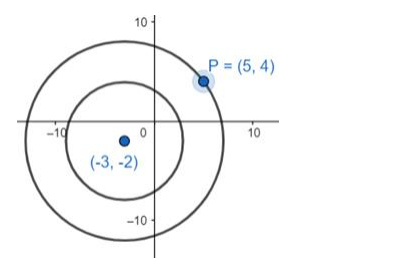# Find the equation of the circle concentric with the circleQuestion:

Find the equation of the circle concentric with the circle $x^{2}+y^{2}+4 x+6 y+11$ = 0 and passing through the point P(5, 4).

Solution:

2 or more circles are said to be concentric if they have the same centre and different radii.Given, $x^{2}+y^{2}+4 x+6 y+11=0$

The concentric circle will have the equation

$x^{2}+y^{2}+4 x+6 y+c^{\prime}=0$

As it passes through P(5, 4), putting this in the equation

$5^{2}+4^{2}+4 \times 5+6 \times 4+c^{\prime}=0$

$\Rightarrow 25+16+20+24+c^{\prime}=0$

$\Rightarrow c^{\prime}=-85$

The required equation is

$x^{2}+y^{2}+4 x+6 y-85=0$